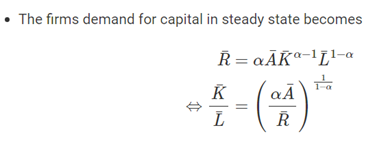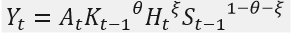Hi Dear All
I have a problem computing and calibrating the steady state.Thank you in advance for your help. I can understand how to calibrate the RBC model. In these models, labor plays an important role. So that by calculating K/L (In firm demand for capital) and labor (in FOC wrt L in household sector) we can proceed well.But in my model, FOC wrt K (in the firm sector) is as follows:For ease of work, I divided all the variables of model (in all equations) on Y.So we have for the above equations:

And I did that for all the equations. But I have a problem only at one point in the model: FOC wrt H (or L (labor)) in the household sector:The problem is that labor can no longer be divided into Y(On the left side of the equation) . That is, we do not have H/Y, but we have H(0.33). But in other equations we have H/Y. For example, in the production function and any equation that has H
My production function is as followsDo I have to change my calibration method or is there a better solution?

It seem this is a model where you cannot analytically solve for the labor to output ratio. You may need a different approach, e…g fixing labor and then computing the parameters to make that work or numerically solve for the ratio.